# Spearman's rank correlation coefficient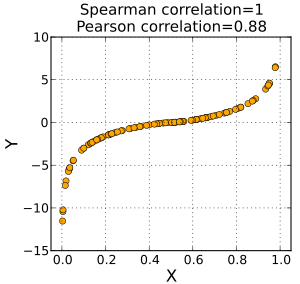A Spearman correlation of 1 results when the two variables being compared are monotonically related, even if their relationship is not linear. This means that all data-points with greater x-values than that of a given data-point will have greater y-values as well. In contrast, this does not give a perfect Pearson correlation.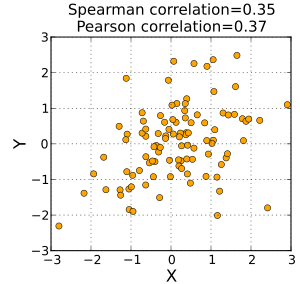When the data are roughly elliptically distributed and there are no prominent outliers, the Spearman correlation and Pearson correlation give similar values.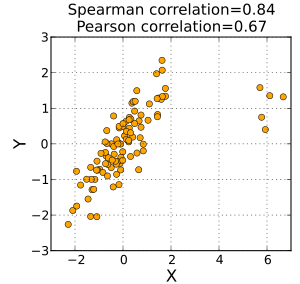The Spearman correlation is less sensitive than the Pearson correlation to strong outliers that are in the tails of both samples. That is because Spearman's rho limits the outlier to the value of its rank.

In statistics, Spearman's rank correlation coefficient or Spearman's rho, named after Charles Spearman and often denoted by the Greek letter(rho) or as, is a nonparametric measure of rank correlation (statistical dependence between the ranking of two variables). It assesses how well the relationship between two variables can be described using a monotonic function.

The Spearman correlation between two variables is equal to the Pearson correlation between the rank values of those two variables; while Pearson's correlation assesses linear relationships, Spearman's correlation assesses monotonic relationships (whether linear or not). If there are no repeated data values, a perfect Spearman correlation of +1 or −1 occurs when each of the variables is a perfect monotone function of the other.

Intuitively, the Spearman correlation between two variables will be high when observations have a similar (or identical for a correlation of 1) rank (i.e. relative position label of the observations within the variable: 1st, 2nd, 3rd, etc.) between the two variables, and low when observations have a dissimilar (or fully opposed for a correlation of -1) rank between the two variables.

Spearman's coefficient is appropriate for both continuous and discrete variables, including ordinal variables. Both Spearman'sand Kendall'scan be formulated as special cases of a more general correlation coefficient.

## Definition and calculation

The Spearman correlation coefficient is defined as the Pearson correlation coefficient between the ranked variables.

For a sample of size n, the n raw scoresare converted to ranks, andis computed from:where
•denotes the usual Pearson correlation coefficient, but applied to the rank variables.
•is the covariance of the rank variables.
•andare the standard deviations of the rank variables.

Only if all n ranks are distinct integers, it can be computed using the popular formulawhere
•, is the difference between the two ranks of each observation.
• n is the number of observations

Identical values are usually each assigned fractional ranks equal to the average of their positions in the ascending order of the values, which is equivalent to averaging over all possible permutations.

If ties are present in the data set, this equation yields incorrect results: Only if in both variables all ranks are distinct, then(cf. tetrahedral number). The first equation—normalizing by the standard deviation—may even be used even when ranks are normalized to [0;1] ("relative ranks") because it is insensitive both to translation and linear scaling.

This method should also not be used in cases where the data set is truncated; that is, when the Spearman correlation coefficient is desired for the top X records (whether by pre-change rank or post-change rank, or both), the user should use the Pearson correlation coefficient formula given above.

The standard error of the coefficient (σ) was determined by Pearson in 1907 and Gosset in 1920. It isThere are several other numerical measures that quantify the extent of statistical dependence between pairs of observations. The most common of these is the Pearson product-moment correlation coefficient, which is a similar correlation method to Spearman's rank, that measures the “linear” relationships between the raw numbers rather than between their ranks.

An alternative name for the Spearman rank correlation is the “grade correlation”; in this, the “rank” of an observation is replaced by the “grade”. In continuous distributions, the grade of an observation is, by convention, always one half less than the rank, and hence the grade and rank correlations are the same in this case. More generally, the “grade” of an observation is proportional to an estimate of the fraction of a population less than a given value, with the half-observation adjustment at observed values. Thus this corresponds to one possible treatment of tied ranks. While unusual, the term “grade correlation” is still in use.

## Interpretation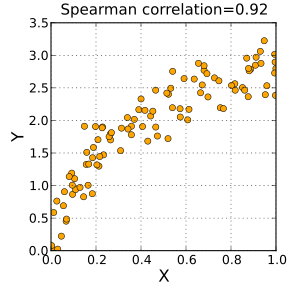A positive Spearman correlation coefficient corresponds to an increasing monotonic trend between X and Y.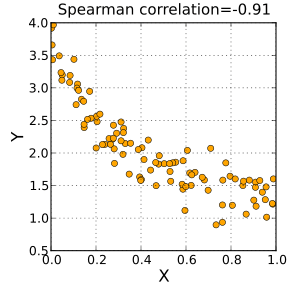A negative Spearman correlation coefficient corresponds to a decreasing monotonic trend between X and Y.

The sign of the Spearman correlation indicates the direction of association between X (the independent variable) and Y (the dependent variable). If Y tends to increase when X increases, the Spearman correlation coefficient is positive. If Y tends to decrease when X increases, the Spearman correlation coefficient is negative. A Spearman correlation of zero indicates that there is no tendency for Y to either increase or decrease when X increases. The Spearman correlation increases in magnitude as X and Y become closer to being perfect monotone functions of each other. When X and Y are perfectly monotonically related, the Spearman correlation coefficient becomes 1. A perfect monotone increasing relationship implies that for any two pairs of data values Xi, Yi and Xj, Yj, that XiXj and YiYj always have the same sign. A perfect monotone decreasing relationship implies that these differences always have opposite signs.

The Spearman correlation coefficient is often described as being "nonparametric". This can have two meanings: First, a perfect Spearman correlation results when X and Y are related by any monotonic function. Contrast this with the Pearson correlation, which only gives a perfect value when X and Y are related by a linear function. The other sense in which the Spearman correlation is nonparametric in that its exact sampling distribution can be obtained without requiring knowledge (i.e., knowing the parameters) of the joint probability distribution of X and Y.

## Example

In this example, the raw data in the table below is used to calculate the correlation between the IQ of a person with the number of hours spent in front of TV per week.

IQ,Hours of TV per week,106 7
86 0
100 27
101 50
99 28
103 29
97 20
113 12
112 6
110 17

Firstly, evaluate. To do so use the following steps, reflected in the table below.

1. Sort the data by the first column (). Create a new columnand assign it the ranked values 1,2,3,...n.
2. Next, sort the data by the second column (). Create a fourth columnand similarly assign it the ranked values 1,2,3,...n.
3. Create a fifth columnto hold the differences between the two rank columns (and).
4. Create one final columnto hold the value of columnsquared.
IQ,Hours of TV per week,rankrank86 0 1 1 0 0
97 20 2 6 −4 16
99 28 3 8 −5 25
100 27 4 7 −3 9
101 50 5 10 −5 25
103 29 6 9 −3 9
106 7 7 3 4 16
110 17 8 5 3 9
112 6 9 2 7 49
113 12 10 4 6 36

Withfound, add them to find. The value of n is 10. These values can now be substituted back into the equation:to givewhich evaluates to ρ = -29/165 = −0.175757575... with a P-value = 0.627188 (using the t distribution)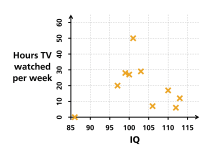Chart of the data presented. It can be seen that there might be a negative correlation, but that the relationship does not appear definitive.

This low value shows that the correlation between IQ and hours spent watching TV is very low, although the negative value suggests that the longer the time spent watching television the lower the IQ. In the case of ties in the original values, this formula should not be used; instead, the Pearson correlation coefficient should be calculated on the ranks (where ties are given ranks, as described above).

## Determining significance

One approach to test whether an observed value of ρ is significantly different from zero (r will always maintain −1 ≤ r ≤ 1) is to calculate the probability that it would be greater than or equal to the observed r, given the null hypothesis, by using a permutation test. An advantage of this approach is that it automatically takes into account the number of tied data values there are in the sample, and the way they are treated in computing the rank correlation.

Another approach parallels the use of the Fisher transformation in the case of the Pearson product-moment correlation coefficient. That is, confidence intervals and hypothesis tests relating to the population value ρ can be carried out using the Fisher transformation:If F(r) is the Fisher transformation of r, the sample Spearman rank correlation coefficient, and n is the sample size, thenis a z-score for r which approximately follows a standard normal distribution under the null hypothesis of statistical independence (ρ = 0).

One can also test for significance usingwhich is distributed approximately as Student's t distribution with n − 2 degrees of freedom under the null hypothesis. A justification for this result relies on a permutation argument.

pvrank is a very recent R package that computes rank correlations and their p-values with various options for tied ranks. It is possible to compute exact Spearman coefficient test p-values for n ≤ 26.

A generalization of the Spearman coefficient is useful in the situation where there are three or more conditions, a number of subjects are all observed in each of them, and it is predicted that the observations will have a particular order. For example, a number of subjects might each be given three trials at the same task, and it is predicted that performance will improve from trial to trial. A test of the significance of the trend between conditions in this situation was developed by E. B. Page and is usually referred to as Page's trend test for ordered alternatives.

## Correspondence analysis based on Spearman's rho

Classic correspondence analysis is a statistical method that gives a score to every value of two nominal variables. In this way the Pearson correlation coefficient between them is maximized.

There exists an equivalent of this method, called grade correspondence analysis, which maximizes Spearman's rho or Kendall's tau.

1. Scale types
2. Lehman, Ann (2005). Jmp For Basic Univariate And Multivariate Statistics: A Step-by-step Guide. Cary, NC: SAS Press. p. 123. ISBN 1-59047-576-3.
3. Myers, Jerome L.; Well, Arnold D. (2003). Research Design and Statistical Analysis (2nd ed.). Lawrence Erlbaum. p. 508. ISBN 0-8058-4037-0.
4. Yule, G.  U.; Kendall, M.  G. (1968) . An Introduction to the Theory of Statistics (14th ed.). Charles Griffin & Co. p. 268.
5. Piantadosi, J.; Howlett, P.; Boland, J. (2007). "Matching the grade correlation coefficient using a copula with maximum disorder". Journal of Industrial and Management Optimization. 3 (2): 305–312.
6. Choi, S. C. (1977). "Tests of Equality of Dependent Correlation Coefficients". Biometrika. 64 (3): 645–647. doi:10.1093/biomet/64.3.645.
7. Fieller, E. C.; Hartley, H. O.; Pearson, E. S. (1957). "Tests for rank correlation coefficients. I". Biometrika. 44: 470–481. doi:10.1093/biomet/44.3-4.470.
8. Press; Vettering; Teukolsky; Flannery (1992). Numerical Recipes in C: The Art of Scientific Computing (2nd ed.). p. 640.
9. Kendall, M. G.; Stuart, A. (1973). The Advanced Theory of Statistics, Volume 2: Inference and Relationship. Griffin. ISBN 0-85264-215-6. (Sections 31.19, 31.21)
10. Amerise, I.L.; Marozzi, M.; Tarsitano, A. "R package pvrank".
11. Page, E. B. (1963). "Ordered hypotheses for multiple treatments: A significance test for linear ranks". Journal of the American Statistical Association. 58 (301): 216–230. doi:10.2307/2282965.
12. Kowalczyk, T.; Pleszczyńska, E.; Ruland, F., eds. (2004). Grade Models and Methods for Data Analysis with Applications for the Analysis of Data Populations. Studies in Fuzziness and Soft Computing. 151. Berlin Heidelberg New York: Springer Verlag. ISBN 978-3-540-21120-4.
• Corder, G.W. & Foreman, D.I. (2014). Nonparametric Statistics: A Step-by-Step Approach, Wiley. ISBN 978-1118840313.
• Daniel, Wayne W. (1990). "Spearman rank correlation coefficient". Applied Nonparametric Statistics (2nd ed.). Boston: PWS-Kent. pp. 358–365. ISBN 0-534-91976-6.
• Spearman C (1904). "The proof and measurement of association between two things". American Journal of Psychology. 15: 72–101. doi:10.2307/1412159.
• Bonett DG, Wright, TA (2000). "Sample size requirements for Pearson, Kendall, and Spearman correlations". Psychometrika. 65: 23–28. doi:10.1007/bf02294183.
• Kendall MG (1970). Rank correlation methods (4th ed.). London: Griffin. ISBN 978-0-852-6419-96. OCLC 136868.
• Hollander M, Wolfe DA (1973). Nonparametric statistical methods. New York: Wiley. ISBN 978-0-471-40635-8. OCLC 520735.
• Caruso JC, Cliff N (1997). "Empirical size, coverage, and power of confidence intervals for Spearman's Rho". Educational and Psychological Measurement. 57: 637–654. doi:10.1177/0013164497057004009.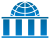Wikiversity has learning materials about Spearman's rank correlation coefficient